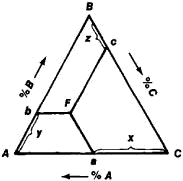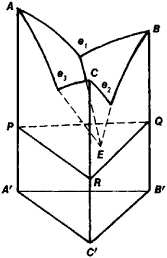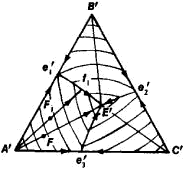# Ternary System

## ternary system

[′tər·nə·rē ′sis·təm]
(chemistry)
Any system with three nonreactive components; in liquid systems, the components may or may not be partially soluble.
McGraw-Hill Dictionary of Scientific & Technical Terms, 6E, Copyright © 2003 by The McGraw-Hill Companies, Inc.
The following article is from The Great Soviet Encyclopedia (1979). It might be outdated or ideologically biased.

## Ternary System

a physicochemical system consisting of three components. Examples of ternary systems with practical importance are metal alloys, molten salts, oxides (slags), and sulfides (mattes), and systems of water and two salts with a common ion. According to the phase rule, the variance, or number of thermodynamic degrees of freedom, of condensed ternary systems (those not containing a gaseous phase) at constant pressure is determined from the formula v = 4 – φ, where φ is the number of phases in the system. Knowledge of the phase diagrams and composition-properties diagrams of ternary systems is necessary for an understanding of the nature of the interaction of the components and the practical use of such systems.

At constant pressure, the state of a ternary system is unambiguously defined by three variables: the temperature T and the concentrations of two of the system’s components. (The concentration of the third component is determined from the condition x + y + z = 100, where x, y, and z are the concentrations of the components.) Concentrations are usually expressed in atomic, molecular, or weight percentages. Thus, a three-dimensional space is required for the construction of phase diagrams of ternary systems: two dimensions serve to indicate changes in composition, and the third indicates the change in temperature of the phase transformations or properties. The temperature or the magnitude of a property is plotted on the vertical axis, and an equilateral triangle, called a concentration triangle (Figure 1), is usually used to indicate the composition of a ternary system. The vertexes A, B, and C of the triangle correspond to the pure components A, B, and C. Each side of the triangle is divided into 100 equal parts. The compositions of the binary systems A-B, B-C, and A-C are given by points on the sides AB, BC, and AC, and the compositions of the ternary system are given by points such as F within the triangle ABC. The method for determining the composition at a point F is based on the properties of equilateral triangles. For example, the lines Fa, Fb, and Fc, which are parallel to sides BC, AC, and AB, respectively, intersect segments Ca, Ab, and Be, whose sum is equal to the side of the triangle. Point Fin Figure 1 corresponds to x percent A, y percent B, and z percent C.Figure 1

Three-dimensional phase diagrams of ternary systems are given as trihedral prisms, bounded from above by complex liquidus surfaces, which are the geometric locations of points corresponding to the temperature of the onset of crystallization. The simplest example of a phase diagram of an A-B-C ternary system is shown in Figure 2. The components of the system do not form chemical compounds with one another, are mutually infinitely miscible in the liquid state, and are incapable of polymorphic transformations. The binary A-B, B-C, and A-C systems, with eutectic points e1, e2, and e3, are represented by the edges of the prism. The liquidus consists of the surfaces Ae1Ee3, Be1Ee2, and Ce2Ee3, representing the onset of crystallization of A, B, and C, respectively. The plane PQR, which passes through the triple eutectic point E and is parallel to the base of the prism, is the soli-dus of the ternary system—the geometric locus of points corresponding to the temperatures of the end of crystallization.

At point E, the number of coexisting phases that is maximal for ternary systems is 4 (liquid and the crystals of A, B, and C), and the phase equilibrium is invariant; that is, the temperature of crystallization and phase composition are constant.

The use of the volumetric representation of the state diagramFigure 2

of a ternary system is very inconvenient in practice; therefore, orthogonal projections and cross sections (horizontal isothermal sections and vertical polythermal sections) are used. The projection of the diagram in Figure 2 onto the plane of the triangle ABC is shown in Figure 3. On this plane, the three liquidus surfaces are represented by three crystallization fields, Ae1Ee3, Be1Ee2, and Ce2Ee3; obviously, the projection of the solidus coincides with the triangle ABC. The arrows indicate the direction of decreasing temperature.

Let us examine the sequence of separation of solid phases in the Ae1Ee3 field. If point Flies on the line AE′, then crystals of A separate from the liquid phase upon cooling, whereas the ratio of the concentrations of B and C remains constant. As a result, when the composition of the ternary system reaches point E′, the combined crystallization of A, B, and C begins at a constant temperature, since a ternary system is invariant for four phases and constant pressure. If the point F1 lies in the region Ae1E′, then crystals of A are the first to separate out, followed by the combined crystallization of A and B along the curve e1E′ when the composition of the liquid phase reaches point f1; solidification is completed at the point E’. Thus, the sequence of crystallization of a liquid phase of composition F1 is shown, in the aggregate, by the segment F1f1E′. Similarly, the course of crystallization of any liquid phase of the A-B-C system may be traced. Isotherms of the onset of crystallization (shown by the fine lines) are also drawn on this projection.

Vertical sections are more complex than diagrams of binary systems, except for the “quasi-binary” sections of ternary systems in which binary and ternary compounds of constant composition are formed. The rules for producing such sections (singular triangulation of ternary systems), which were first formulated in 1925 by N. S. Kurnakov, simplify the examination of complex ternary systems.Figure 3

The experimental construction of complete phase diagrams of ternary systems is very laborious. However, the construction of side binary systems and the location of the monovariant curves, invariant points, and areas of solid solutions based on the components of a ternary system are frequently sufficient for practical purposes. In some cases, thermodynamic calculations of very simple types of binary and ternary phase diagrams give results that are close to experimental data. Various simplified models are used for the analysis of equilibria in ternary systems; special programs have been developed and computer technology has been used to solve complex thermodynamic equations.

### REFERENCES

Kurnakov, N. S. Izbr. trudy, vols. 1–3. Moscow, 1960–63.
Anosov, V. Ia., and S. A. Pogodin. Osnovnye nachala fizikokhimicheskogo analiza. Moscow-Leningrad, 1947.
Volovik, B. E., and M. V. Zakharov. Troinye i chetvernye sistemy. Moscow, 1948.
Petrov, D. A. Troinye sistemy. Moscow, 1953.
Spravochnik po plavkosti sistem iz bezvodnykh neorganicheskikh solei, vols. 1–2. Moscow-Leningrad, 1961.
Zakharov, A. M. Diagrammy sostoianii dvoinykh i troinykh sistem. Moscow, 1964.
Vaniukov, A. V., and V. Ia. Zaitsev. Shlaki i shteiny tsvetnoi metallurgii. Moscow, 1969.
Krestovnikov, A. N., and V. N. Vigdoroyich. Khimicheskaia termodinamika, 2nd ed. Moscow, 1973.
Kaufman, L., and H. Bernstein. Raschet diagramm sostoianiia s pomoshch’iu EVM. Moscow, 1972. (Translated from English.)
Diagrammy sostoianiia metallicheskikh sistem, fases. 1–18. Moscow, 1959–75.
References in periodicals archive ?
The zeta potential of Gt was slightly higher in the ternary system than in the binary Gt-IHP system, indicating that the presence of Zn(II) reduced the negative charge on the Gt surface.
Yen, "The 260[degrees]C phase equilibria of the Sn-Sb-Cu ternary system and interfacial reactions at the Sn-Sb/Cu joints," Intermetallics, vol.
 published the ternary system (NaCl + [C.sub.2][H.sub.5]OH + [H.sub.2]O) equilibrium at 293.15 K.
 studied the thermal stability of a novel eutectic ternary system by placing the salt mixture in an argon atmosphere with a constant heating rate.
Among these, the B/SBS/MMT (OMMT) ternary system is the most studied one.
In the SHC ternary system, the values of pH are higher (comparing Ca[(OH).sub.2] contents in CFB and PF systems) due to the proton capture of sulfides, releasing the O[H.sup.-] ions into the solution (Eqs.
In current analysis the addition of [Pr.sub.6][O.sub.11] to the ternary system Sn[O.sub.2].1.0%CoO.0.05%[Ta.sub.2][O.sub.5] verified the influence of this oxide in potential barrier formation and in microstructure modifications.
RMSEC refers to the uncertainty of calibration for the selected ternary system; a small value of RMSEC indicates that the calibration has less error.
Therefore, our work shed light on the influence of the ternary system LDH-Pd-CD on the Suzuki reaction under mild conditions applying aqueous medium.
(17) compared the 1100 [degrees]C isothermal section of the Co-Mo-Ni ternary system which was constructed using the equilibrated alloys approach (Fig.

Site: Follow: Share:
Open / Close